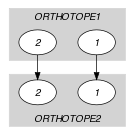## 5.411. two_orth_include

Origin

Used for defining $\mathrm{𝚍𝚒𝚏𝚏𝚗}_\mathrm{𝚒𝚗𝚌𝚕𝚞𝚍𝚎}$.

Constraint

$\mathrm{𝚝𝚠𝚘}_\mathrm{𝚘𝚛𝚝𝚑}_\mathrm{𝚒𝚗𝚌𝚕𝚞𝚍𝚎}\left(\mathrm{𝙾𝚁𝚃𝙷𝙾𝚃𝙾𝙿𝙴}\mathtt{1},\mathrm{𝙾𝚁𝚃𝙷𝙾𝚃𝙾𝙿𝙴}\mathtt{2},\mathrm{𝙳𝙸𝙼}\right)$

Type
 $\mathrm{𝙾𝚁𝚃𝙷𝙾𝚃𝙾𝙿𝙴}$ $\mathrm{𝚌𝚘𝚕𝚕𝚎𝚌𝚝𝚒𝚘𝚗}\left(\mathrm{𝚘𝚛𝚒}-\mathrm{𝚍𝚟𝚊𝚛},\mathrm{𝚜𝚒𝚣}-\mathrm{𝚍𝚟𝚊𝚛},\mathrm{𝚎𝚗𝚍}-\mathrm{𝚍𝚟𝚊𝚛}\right)$
Arguments
 $\mathrm{𝙾𝚁𝚃𝙷𝙾𝚃𝙾𝙿𝙴}\mathtt{1}$ $\mathrm{𝙾𝚁𝚃𝙷𝙾𝚃𝙾𝙿𝙴}$ $\mathrm{𝙾𝚁𝚃𝙷𝙾𝚃𝙾𝙿𝙴}\mathtt{2}$ $\mathrm{𝙾𝚁𝚃𝙷𝙾𝚃𝙾𝙿𝙴}$ $\mathrm{𝙳𝙸𝙼}$ $\mathrm{𝚒𝚗𝚝}$
Restrictions
 $|\mathrm{𝙾𝚁𝚃𝙷𝙾𝚃𝙾𝙿𝙴}|>0$ $\mathrm{𝚛𝚎𝚚𝚞𝚒𝚛𝚎}_\mathrm{𝚊𝚝}_\mathrm{𝚕𝚎𝚊𝚜𝚝}$$\left(2,\mathrm{𝙾𝚁𝚃𝙷𝙾𝚃𝙾𝙿𝙴},\left[\mathrm{𝚘𝚛𝚒},\mathrm{𝚜𝚒𝚣},\mathrm{𝚎𝚗𝚍}\right]\right)$ $\mathrm{𝙾𝚁𝚃𝙷𝙾𝚃𝙾𝙿𝙴}.\mathrm{𝚜𝚒𝚣}\ge 0$ $\mathrm{𝙾𝚁𝚃𝙷𝙾𝚃𝙾𝙿𝙴}.\mathrm{𝚘𝚛𝚒}\le \mathrm{𝙾𝚁𝚃𝙷𝙾𝚃𝙾𝙿𝙴}.\mathrm{𝚎𝚗𝚍}$ $|\mathrm{𝙾𝚁𝚃𝙷𝙾𝚃𝙾𝙿𝙴}\mathtt{1}|=|\mathrm{𝙾𝚁𝚃𝙷𝙾𝚃𝙾𝙿𝙴}\mathtt{2}|$ $\mathrm{𝚘𝚛𝚝𝚑}_\mathrm{𝚕𝚒𝚗𝚔}_\mathrm{𝚘𝚛𝚒}_\mathrm{𝚜𝚒𝚣}_\mathrm{𝚎𝚗𝚍}$$\left(\mathrm{𝙾𝚁𝚃𝙷𝙾𝚃𝙾𝙿𝙴}\mathtt{1}\right)$ $\mathrm{𝚘𝚛𝚝𝚑}_\mathrm{𝚕𝚒𝚗𝚔}_\mathrm{𝚘𝚛𝚒}_\mathrm{𝚜𝚒𝚣}_\mathrm{𝚎𝚗𝚍}$$\left(\mathrm{𝙾𝚁𝚃𝙷𝙾𝚃𝙾𝙿𝙴}\mathtt{2}\right)$ $\mathrm{𝙳𝙸𝙼}>0$ $\mathrm{𝙳𝙸𝙼}\le |\mathrm{𝙾𝚁𝚃𝙷𝙾𝚃𝙾𝙿𝙴}\mathtt{1}|$
Purpose

Let ${P}_{1}$ and ${P}_{2}$ respectively denote the projections of $\mathrm{𝙾𝚁𝚃𝙷𝙾𝚃𝙾𝙿𝙴}\mathtt{1}$ and $\mathrm{𝙾𝚁𝚃𝙷𝙾𝚃𝙾𝙿𝙴}\mathtt{2}$ onto dimension $\mathrm{𝙳𝙸𝙼}$. If ${P}_{1}$ and ${P}_{2}$ overlap then, either ${P}_{1}$ is included in ${P}_{2}$, either ${P}_{2}$ is included in ${P}_{1}$.

Example
$\left(\begin{array}{c}〈\mathrm{𝚘𝚛𝚒}-1\mathrm{𝚜𝚒𝚣}-3\mathrm{𝚎𝚗𝚍}-4,\mathrm{𝚘𝚛𝚒}-1\mathrm{𝚜𝚒𝚣}-1\mathrm{𝚎𝚗𝚍}-2〉,\hfill \\ 〈\mathrm{𝚘𝚛𝚒}-1\mathrm{𝚜𝚒𝚣}-2\mathrm{𝚎𝚗𝚍}-3,\mathrm{𝚘𝚛𝚒}-2\mathrm{𝚜𝚒𝚣}-3\mathrm{𝚎𝚗𝚍}-5〉,1\hfill \end{array}\right)$
##### Figure 5.411.1. Initial and final graph of the $\mathrm{𝚝𝚠𝚘}_\mathrm{𝚘𝚛𝚝𝚑}_\mathrm{𝚒𝚗𝚌𝚕𝚞𝚍𝚎}$ constraint(a) (b)
Typical
$|\mathrm{𝙾𝚁𝚃𝙷𝙾𝚃𝙾𝙿𝙴}|>1$
Symmetry

Arguments are permutable w.r.t. permutation $\left(\mathrm{𝙾𝚁𝚃𝙷𝙾𝚃𝙾𝙿𝙴}\mathtt{1},\mathrm{𝙾𝚁𝚃𝙷𝙾𝚃𝙾𝙿𝙴}\mathtt{2}\right)$ $\left(\mathrm{𝙳𝙸𝙼}\right)$.

Used in

related: $\mathrm{𝚍𝚒𝚏𝚏𝚗}$ (an extension of the $\mathrm{𝚍𝚒𝚏𝚏𝚗}$ constraint).

Keywords
Arc input(s)

$\mathrm{𝙾𝚁𝚃𝙷𝙾𝚃𝙾𝙿𝙴}\mathtt{1}$ $\mathrm{𝙾𝚁𝚃𝙷𝙾𝚃𝙾𝙿𝙴}\mathtt{2}$

Arc generator
$\mathrm{𝑃𝑅𝑂𝐷𝑈𝐶𝑇}$$\left(=\right)↦\mathrm{𝚌𝚘𝚕𝚕𝚎𝚌𝚝𝚒𝚘𝚗}\left(\mathrm{𝚘𝚛𝚝𝚑𝚘𝚝𝚘𝚙𝚎}\mathtt{1},\mathrm{𝚘𝚛𝚝𝚑𝚘𝚝𝚘𝚙𝚎}\mathtt{2}\right)$

Arc arity
Arc constraint(s)
$\begin{array}{c}\bigwedge \left(\begin{array}{c}\mathrm{𝚘𝚛𝚝𝚑𝚘𝚝𝚘𝚙𝚎}\mathtt{1}.\mathrm{𝚔𝚎𝚢}=\mathrm{𝙳𝙸𝙼},\hfill \\ \mathrm{𝚘𝚛𝚝𝚑𝚘𝚝𝚘𝚙𝚎}\mathtt{1}.\mathrm{𝚘𝚛𝚒}<\mathrm{𝚘𝚛𝚝𝚑𝚘𝚝𝚘𝚙𝚎}\mathtt{2}.\mathrm{𝚎𝚗𝚍},\hfill \\ \mathrm{𝚘𝚛𝚝𝚑𝚘𝚝𝚘𝚙𝚎}\mathtt{2}.\mathrm{𝚘𝚛𝚒}<\mathrm{𝚘𝚛𝚝𝚑𝚘𝚝𝚘𝚙𝚎}\mathtt{1}.\mathrm{𝚎𝚗𝚍},\hfill \\ \mathrm{𝚘𝚛𝚝𝚑𝚘𝚝𝚘𝚙𝚎}\mathtt{1}.\mathrm{𝚜𝚒𝚣}>0,\hfill \\ \mathrm{𝚘𝚛𝚝𝚑𝚘𝚝𝚘𝚙𝚎}\mathtt{2}.\mathrm{𝚜𝚒𝚣}>0\hfill \end{array}\right)⇒\hfill \\ \begin{array}{c}\begin{array}{c}\mathrm{𝚖𝚒𝚗}\left(\mathrm{𝚘𝚛𝚝𝚑𝚘𝚝𝚘𝚙𝚎}\mathtt{1}.\mathrm{𝚎𝚗𝚍},\mathrm{𝚘𝚛𝚝𝚑𝚘𝚝𝚘𝚙𝚎}\mathtt{2}.\mathrm{𝚎𝚗𝚍}\right)-\hfill \\ \mathrm{𝚖𝚊𝚡}\left(\mathrm{𝚘𝚛𝚝𝚑𝚘𝚝𝚘𝚙𝚎}\mathtt{1}.\mathrm{𝚘𝚛𝚒},\mathrm{𝚘𝚛𝚝𝚑𝚘𝚝𝚘𝚙𝚎}\mathtt{2}.\mathrm{𝚘𝚛𝚒}\right)\hfill \end{array}=\hfill \\ \mathrm{𝚖𝚒𝚗}\left(\mathrm{𝚘𝚛𝚝𝚑𝚘𝚝𝚘𝚙𝚎}\mathtt{1}.\mathrm{𝚜𝚒𝚣},\mathrm{𝚘𝚛𝚝𝚑𝚘𝚝𝚘𝚙𝚎}\mathtt{2}.\mathrm{𝚜𝚒𝚣}\right)\hfill \end{array}\hfill \end{array}$
Graph property(ies)
$\mathrm{𝐍𝐀𝐑𝐂}$$=1$